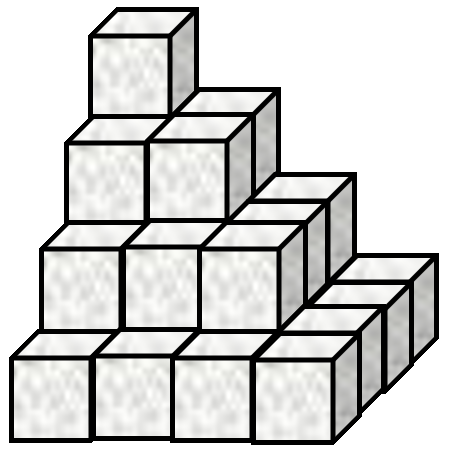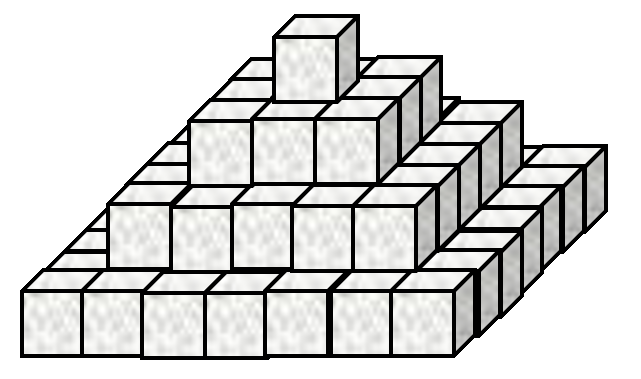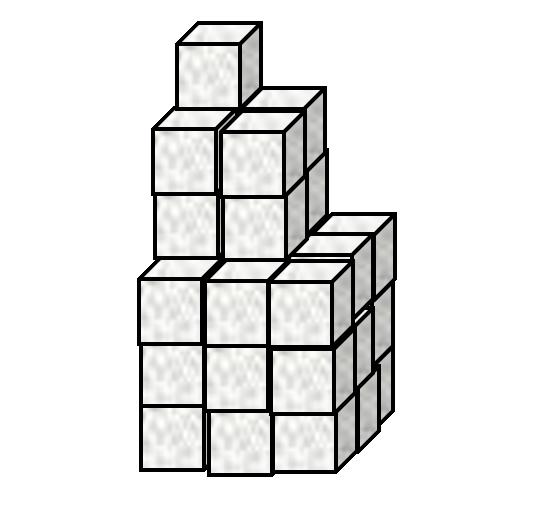It is easy to add lists of numbers together

      1 2 3 + 4 5 6
5 7 9


Negative numbers are written with a high minus ¯ to differentiate between negation ¯3 = -3 and literal negative numbers

      1 2 3 - 1 0 ¯1
0 2 4


### Singleton extension

Dyadic functions (functions with two input arguments, one on the left and one on the right) can map between a single value and an array of values.

      3 × 1 10 100
3 30 300
3 = 1 2 3 4 5
0 0 1 0 0


### The reduction operator

Adding a list of numbers could become very tedious…

      1+2+3+4+5+6+7+8+9+10+11+12+13+14+15
120


The reduce operator / inserts the function to its left between parts of the right argument array.

      +/1 2 3 4 5 6 7 8 9 10 11 12 13 14 15
120


### The index generator

The index generator ⍳ generates integers up to the integer right argument ⍵

      ⍳10
1 2 3 4 5 6 7 8 9 10


So we can do an arithmetic sum as follows

%% \sum_{n=1}^N n %% +/⍳N

### Order of execution

Infix (dyadic) functions have a short left scope and long right scope.

      10×⍳2+5
10 20 30 40 50 60 70


The expresssion above is “ten times the indices from 1 to two plus five, or in short: “ten times iota two plus five”. We can make it clearer using (superfluous) parentheses ().

      10×(⍳(2+5))
10 20 30 40 50 60 70


Of course, we can change the order of execution using different parentheses.

      (10×⍳2)+5
15 25


# Problems Set 1: The APL Pocket Calculator

1. A Mathematical Notation

Use APL to evaluate the following

1. @@479001600 = \prod_{n=1}^{12} n@@ (multiply together the first twelve integers)

2. @@1785 = \sum_{n=1}^{17}n^2@@ (add together the first seventeen squared integers)

3. @@10100 = \sum_{n=1}^{100}2n@@ (add together the first one hundred positive even integers)

4. @@10000 = \sum_{n=1}^{100}2n-1@@ (add together the first one hundred odd integers)

5. In traditional mathematical notation (TMN), the following equation equals 0, why does the following return 70?

       84 - 12 - 1 - 13 - 28 - 9 - 6 - 15
70

2. Pyramid Schemes
1. Sugar cubes are stacked in an arrangement as shown by Figure 1.This stack has 4 layers and a total of 30 cubes. In a similar stack with 467 layers there are 34058310 cubes.

Write an APL expression that calculates the number of cubes at any layer for this arrangement.

2. Now consider the stack in Figure 2.The arrangement in Figure 2 has 4 layers and 84 cubes. In a similar stack with 812 layers there are 713849500 cubes.

Write an APL expression that calculates the number of cubes at any layer for this arrangement.

3. Now look at Figure 3.The stack in Figure 3 has 3 “layers” and 36 cubes in total. In a similar stack with 68 “layers” there are 5503716 cubes.

Write an APL expression that calculates the number of cubes at any layer for this arrangement.

3. What’s in a Vector? ⎕AVU is a list (vector) of numbers (don’t worry about what it represents). Find the following properties of ⎕AVU.
1. Find the sum of all the values in ⎕AVU.

Answer: 646112

2. Find the product of all the values in ⎕AVU.

Answer: 0

3. What is the length of ⎕AVU?

Answer: 256

4. Find the mean average of ⎕AVU.

Answer: 2523.875